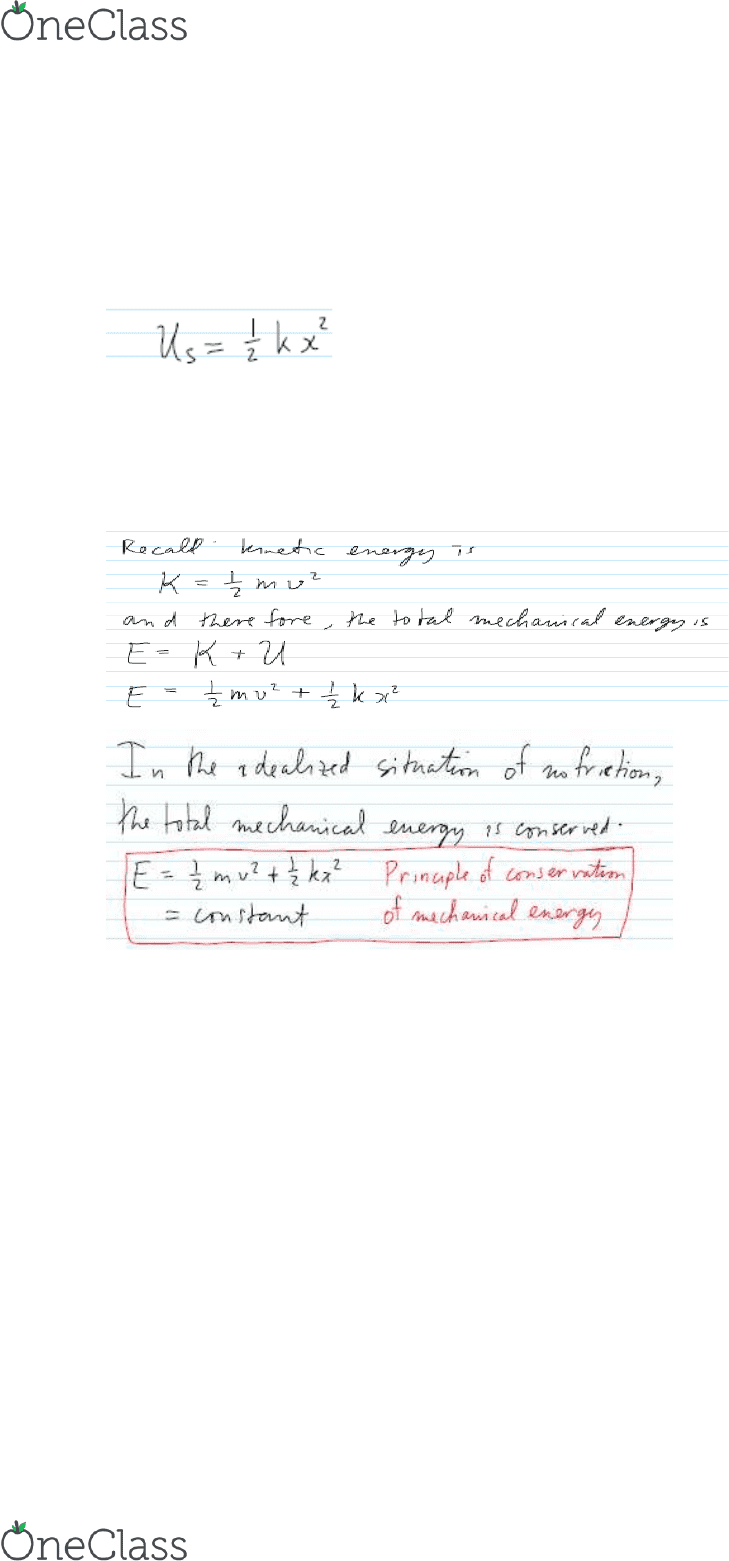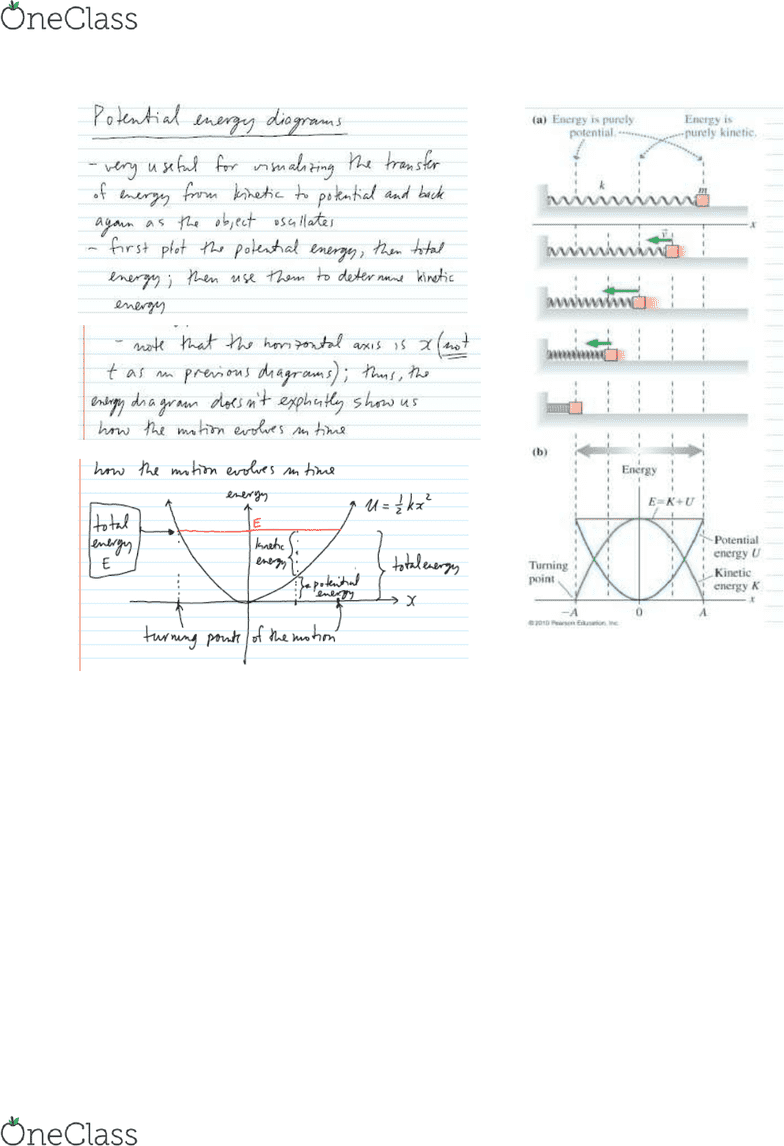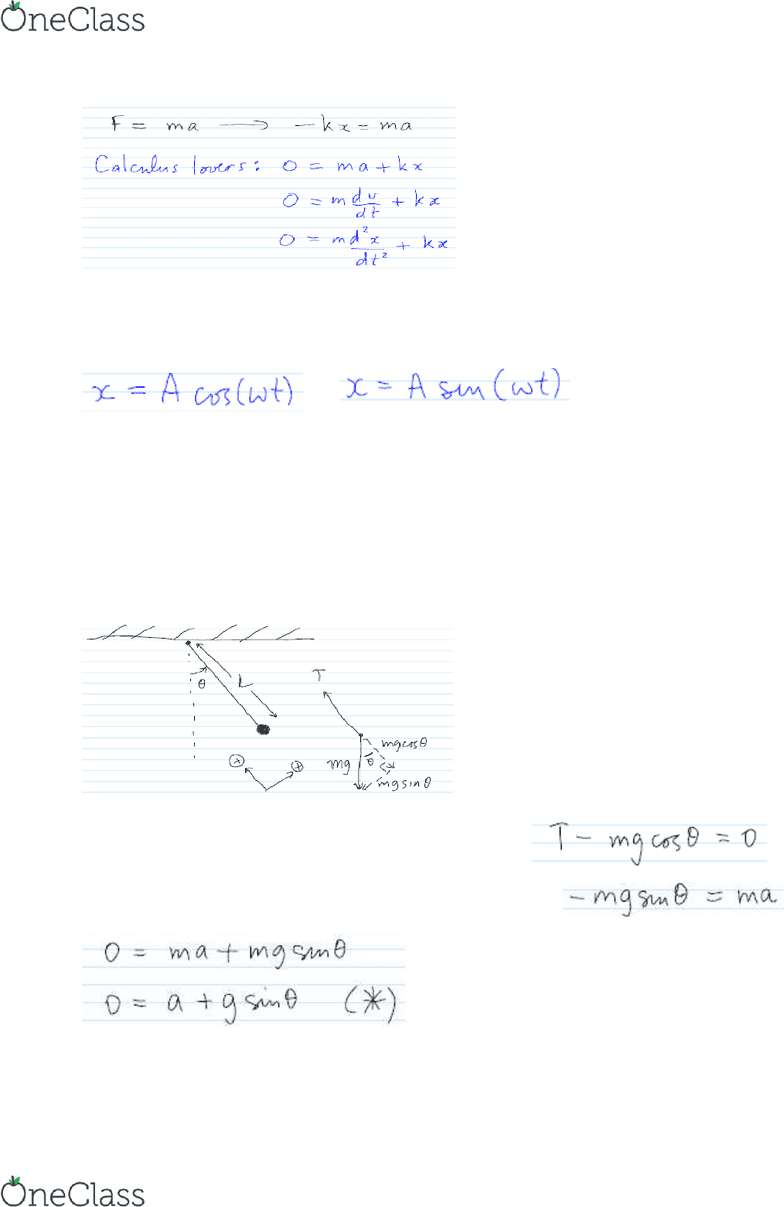# PHYS 1P21 Lecture 46: Elastic potential energy and Damped oscillations

17 views8 pages
School
Department
CourseSimple Harmonic Motion - Elastic potential energy and Damped oscillations
Elastic potential energy
An argument similar in spirit to the one above for gravitational potential energy shows that the
formula for elastic potential energy is (see page 285ff in Section 10.3 of the textbook for details;
calculus lovers, antidifferentiate Hooke's law)
where xis the displacement of the spring from its equilibrium position
and kis the stiffness constant of the spring.
Where is potential energy stored? Answering this question leads to the concept of a force field;
we'll discuss force fields in PHYS
find more resources at oneclass.com
find more resources at oneclass.com
Unlock document

This preview shows pages 1-3 of the document.
Unlock all 8 pages and 3 million more documents.

Already have an account? Log inExercises
8. Consider a block of mass 5.1 kg attached to a spring. The position-time function of this
oscillator is x= 8.2 sin(2.7t), where xis measured in cm and tis measured in seconds.
(a) Determine the total mechanical energy of the oscillator.
(b) Determine the stiffness constant of the spring.
(c) Determine the maximum potential energy.
(d) Determine the maximum kinetic energy.
(e) Determine the positions at which the kinetic energy and the potential energy of the oscillator
are equal.
Pendulum motion
Recall from earlier in these lecture notes that for a block on the end of a spring, applying
Newton's law to the block results in
find more resources at oneclass.com
find more resources at oneclass.com
Unlock document

This preview shows pages 1-3 of the document.
Unlock all 8 pages and 3 million more documents.

Already have an account? Log inThis is an example of a differential equation, and to solve a differential equation means to
determine a position function x(t) that satisfies the equation. You'll learn how to do this in
second-year physics (PHYS 2P20), and second-year math (MATH 2P08), but for now you can
verify that position functions of the form
and
both satisfy the differential equation above, provided that satisfies a certain condition (the same
condition that was observed earlier in the notes).
From a different perspective, we can also infer that if a physical phenomenon is modelled by a
differential equation of the form given above, the phenomenon is an example of simple harmonic
motion.
Let's consider a simple pendulum. First draw a free-body diagram:
In the radial direction, applying Newton's second law gives:
In the tangential direction, applying Newton's second law gives:
find more resources at oneclass.com
find more resources at oneclass.com
Unlock document

This preview shows pages 1-3 of the document.
Unlock all 8 pages and 3 million more documents.

Already have an account? Log in

# Get OneClass Notes+

Unlimited access to class notes and textbook notes.

75% OFF
\$8 USD/m
###### Monthly
\$30 USD/m
You will be charged \$96 USD upfront and auto renewed at the end of each cycle. You may cancel anytime under Payment Settings. For more information, see our Terms and Privacy.
Payments are encrypted using 256-bit SSL. Powered by Stripe.